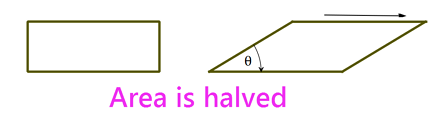# Sliding A Dimension

Geometry Level 1Imagine a rectangle. Keeping the base firm, if you slide the rectangle from the top to make a parallelogram so that its area is exactly half of that of the rectangle (with same dimensions), what would be the smaller angle of that parallelogram in degrees?

×